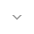# CircularIntArray

``` public final class CircularIntArray ```
``` extends Object ``` ``` ```

 java.lang.Object ↳ android.support.v4.util.CircularIntArray

CircularIntArray is a circular integer array data structure that provides O(1) random read, O(1) prepend and O(1) append. The CircularIntArray automatically grows its capacity when number of added integers is over its capacity.

## Summary

### Public constructors

``` CircularIntArray() ```

Creates a circular array with default capacity.

``` CircularIntArray(int minCapacity) ```

Creates a circular array with capacity for at least `minCapacity` elements.

### Public methods

``` void``` ``` addFirst(int e) ```

Add an integer in front of the CircularIntArray.

``` void``` ``` addLast(int e) ```

Add an integer at end of the CircularIntArray.

``` void``` ``` clear() ```

Remove all integers from the CircularIntArray.

``` int``` ``` get(int n) ```

Get nth (0 <= n <= size()-1) integer of the CircularIntArray.

``` int``` ``` getFirst() ```

Get first integer of the CircularIntArray.

``` int``` ``` getLast() ```

Get last integer of the CircularIntArray.

``` boolean``` ``` isEmpty() ```

Return true if size() is 0.

``` int``` ``` popFirst() ```

Remove first integer from front of the CircularIntArray and return it.

``` int``` ``` popLast() ```

Remove last integer from end of the CircularIntArray and return it.

``` void``` ``` removeFromEnd(int numOfElements) ```

Remove multiple elements from end of the CircularIntArray, ignore when numOfElements is less than or equals to 0.

``` void``` ``` removeFromStart(int numOfElements) ```

Remove multiple integers from front of the CircularIntArray, ignore when numOfElements is less than or equals to 0.

``` int``` ``` size() ```

Get number of integers in the CircularIntArray.

### Inherited methodsFrom class ``` java.lang.Object ```

## Public constructors

### CircularIntArray

`CircularIntArray ()`

Creates a circular array with default capacity.

### CircularIntArray

`CircularIntArray (int minCapacity)`

Creates a circular array with capacity for at least `minCapacity` elements.

Parameters
`minCapacity` `int`: the minimum capacity, between 1 and 2^30 inclusive

## Public methods

`void addFirst (int e)`

Add an integer in front of the CircularIntArray.

Parameters
`e` `int`: Integer to add.

`void addLast (int e)`